# C++ program to convert lowercase to uppercase

In this article, you will learn and get code on lowercase to uppercase conversion of both characters and strings in C++. The program is created with and without using library functions. Here is the list of programs available:

Note: Here, lowercase and uppercase characters actually mean lowercase and uppercase alphabet characters, respectively.

### Lowercase Character

An alphabet character written in a small letter, say "c," is called a lowercase alphabet character. The ASCII values of all the 26 lowercase letters (a–z) are 97–122. That is, the ASCII value of a is 97, b is 98, c is 99, and so on.

### Uppercase Character

An alphabet character written in capital letters, say C, is called an uppercase alphabet. The ASCII values of all the 26 uppercase letters (A–Z) are 65–90. That is, the ASCII value of A is 65, B is 66, C is 67, and so on.

### Lowercase and Uppercase String

If all alphabet characters in a string are written in small letters, then the string can be called a "lowercase string." For example, codescracker. Whereas, if all the alphabetic characters of a string are written in capital letters, then the string can be called an "uppercase string." CODESCRACKER, for example.

## C++ Lowercase character to uppercase

Let's create a program that receives an alphabet character (in lowercase) from the user at run-time. Now convert and print the equivalent uppercase value of the given (entered) character (in lowercase) without using the library function.

The question is: write a program in C++ that converts lowercase characters entered by the user to their equivalent uppercase characters. Here is the answer to this question:

```#include<iostream>
using namespace std;
int main()
{
char chLower, chUpper;
int ascii;
cout<<"Enter the Character: ";
cin>>chLower;
ascii = chLower;
ascii = ascii-32;
chUpper = ascii;
cout<<"\nUppercase: "<<chUpper;
cout<<endl;
return 0;
}```

This program was built and runs under the Code::Blocks IDE. Here is its sample run: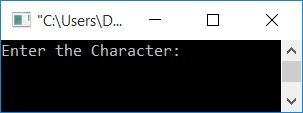Now supply character input (in lowercase), say "c," and press the ENTER key to see its equivalent value in uppercase, as shown in the snapshot given below: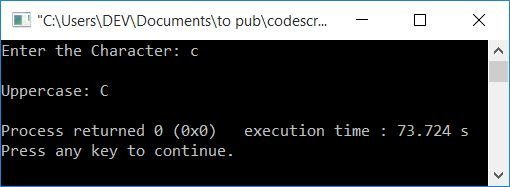From the above program, the statement:

`ascii = chLower;`

The ASCII value of the character stored in chLower was set to ascii. Because chLower is of a char (character) type variable, whereas ascii is an int (integer) type variable, the ASCII value (integer) of chLower gets initialized to ascii.

For example, if the user enters c as a lowercase alphabet character input, then c gets stored in chLower and its ASCII value of 99 gets initialized to ascii.

Note: Because the ASCII value of the capital letter "A" is 65, where the ASCII value of the small letter "a" is 97. The difference between both ASCII values is 32. Therefore, subtracting 32 from the ASCII value of a lowercase alphabet character gives you the ASCII value of the equivalent alphabet character in uppercase.

So the statement,

`ascii = ascii-32;`

initializes ascii-32, 99-32, or 67 to ascii. So the new value of ascii is 67. And 67 is the ASCII value of the capital letter "C."

Therefore, the statement given below:

`chUpper = ascii;`

initializes the character value whose ASCII value is 67. That will be C. As a result, chUpper = C. Now print the value of chUpper.

### What if the user enters an invalid input?

To deal with an invalid input received at runtime by the user, such as:

• a number
• a special character
• or an uppercase character

Then, here is another program to deal with these types of inputs:

```#include<iostream>
using namespace std;
int main()
{
char chLower, chUpper;
int ascii;
cout<<"Enter the Character: ";
cin>>chLower;
if(chLower>='a' && chLower<='z')
{
ascii = chLower;
ascii = ascii-32;
chUpper = ascii;
cout<<endl<<chLower<<" in Uppercase: "<<chUpper;
}
else if(chLower>='A' && chLower<='Z')
else
cout<<"\nInvalid Input!";
cout<<endl;
return 0;
}```

Here is its sample run with user input d: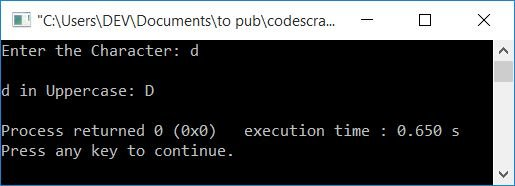Here is another sample run with user input D: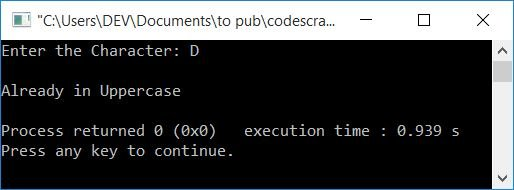And here is the last sample run with user input 3: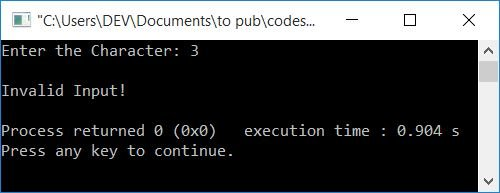## C++ Lowercase string to uppercase

Now let's convert the lowercase string received by the user to its equivalent uppercase string. Because string is a combination of characters, we have to convert all the characters (available in string) one by one to uppercase, as shown in the program given below:

```#include<iostream>
#include<stdio.h>
using namespace std;
int main()
{
char strLower, strUpper;
int ascii, i=0, chk=0;
cout<<"Enter the String: ";
gets(strLower);
while(strLower[i]!='\0')
{
if(strLower[i]>='a' && strLower[i]<='z')
{
ascii = strLower[i];
ascii = ascii-32;
strUpper[i] = ascii;
chk++;
}
else
strUpper[i] = strLower[i];
i++;
}
strUpper[i]='\0';
if(chk==0)
else
cout<<"\nUppercase of String: "<<strUpper;
cout<<endl;
return 0;
}```

Here is its sample run with user input This is CodesCracker:### In C++, use the Library Function strupr() to convert lowercase to uppercase

This is the last program that uses the library function strupr(). The function takes a string as its argument and converts it into its equivalent uppercase string. The function is defined in the string.h header file.

```#include<iostream>
#include<stdio.h>
#include<string.h>
using namespace std;
int main()
{
char str;
cout<<"Enter the String: ";
gets(str);
cout<<"\nUppercase of String: "<<strupr(str);
cout<<endl;
return 0;
}```

This program produces the same output as the previous one.

#### The same program in different languages

C++ Quiz

« Previous Program Next Program »